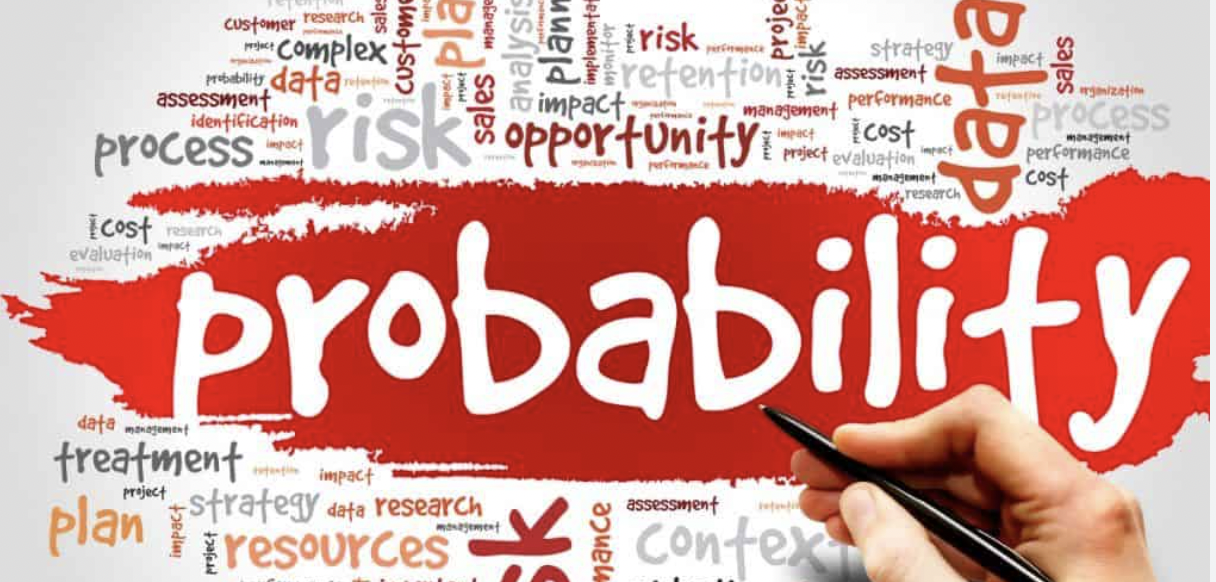# Probability

After watching the probability video, answer the following:

1) What is probability? How would you describe it?

2) What are some examples of probability from real life?

3) What did you learn about probability from the game?